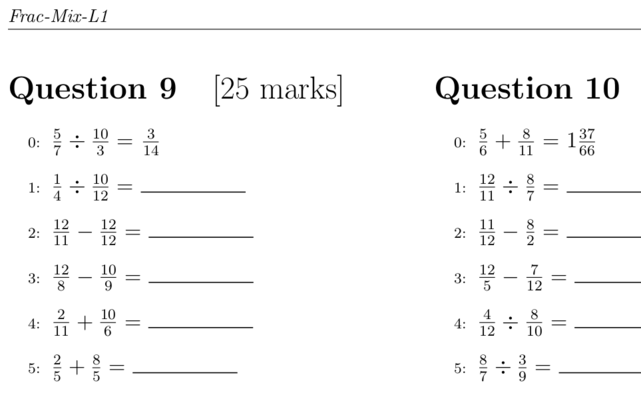# Printable PDF Math Exercises

A while ago I created some software to create printable sheets of math questions. The idea was to have many questions for the kids to practice basic arithmetic with whole numbers, decimals and fractions. Paper-based questions, as opposed to online, were the most appropriate at the time, as it is very quick to give them questions at any time/place. I don't use them much any more, but thought I would put them online if anyone wanted them.There are other such printable worksheets of math questions offered online which are much better, e.g. Math-Aids and Math4Children, and also online questions (after briefly trying my own Moodle questions, we are now using Khan Academy). I created my own as I initially wanted to create a certain set of questions. But then I expanded to cover a few more questions. The "software" is just a poorly designed Bash shell script, which modifies a LaTeX template with random questions, and then produces a PDF of the questions and answers.

The question sheets cover the following (click the link for the individual PDF or download this archive of all the files):

• Addition of positive whole numbers: Q1 A1; Q2 A2
• Subtraction of positive whole numbers: Q1 A1; Q2 A2
• Multiplication of positive whole numbers: Q1 A1; Q2 A2
• Division of positive whole numbers: Q1 A1; Q2 A2
• Addition and subtraction of negative whole numbers: Q1 A1; Q2 A2; Q3 A3
• Multiplication of negative whole numbers: Q A
• Division of negative whole numbers: Q1 A1; Q2 A2
• Quotient and remainder when dividing: Q1 A1; Q2 A2
• Addition and subtraction of decimals: Q A
• Multiplication and division of decimals: Q A
• Prime factors: Q A
• Addition of fractions: Q A
• Subtraction of fractions: Q A
• Multiplication of fractions: Q A
• Division of fractions: Q A
• Mixed operations on fractions: Q A
• Simplifying fractions: Q A
• Converting between fractions and decimals: Q1 A1; Q2 A2

Each PDF file contains either 16 or 24 sheets of questions, which each sheet containing 50 individual questions. The Question sheet has space for writing the answer, and the Answer sheet of course has the answer!